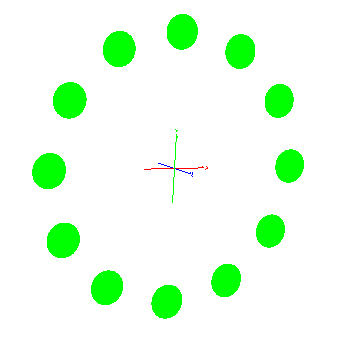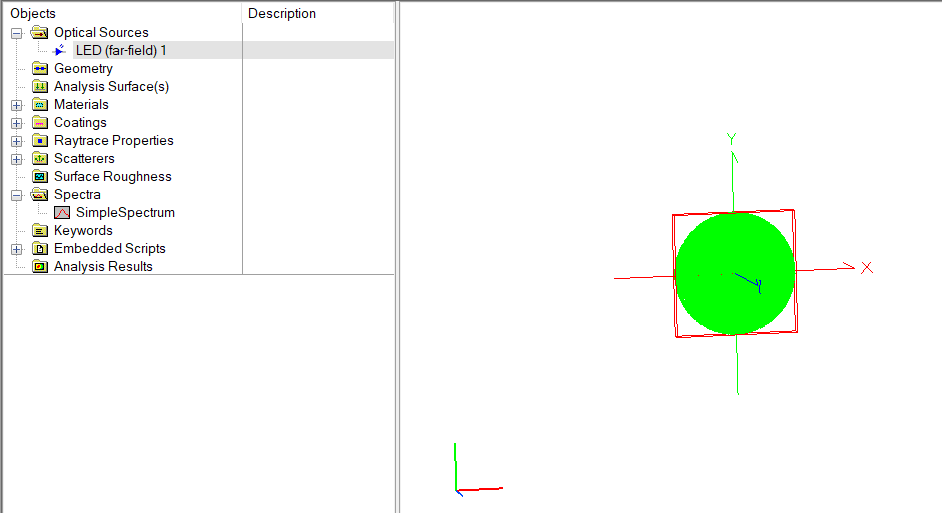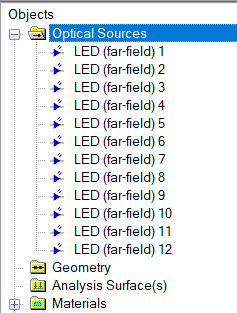# Circular Array of Sources

As of FRED version 18.61 only rectilinear arrays are possible when using the built-in array feature. Therefore placing objects or sources in a circular array needs to be done manually or by using a custom script. This is a simple example.Let's say we want to create a ring of sources in a circular array. Assuming that the total number of sources is reasonably small (i.e. not hundreds or thousands) then a quick way to achieve this is:

1. Create one source manually2. Using the mouse / keyboard, copy/paste this source multiple times until you have the desired number of sources (all located at (0,0,0).3. Create the following script, edit the line that sets the radius ("rad"), and run.

```    'assume all sources are already created
'(create one manually, then copy and paste)

Dim i As Long, xPos As Double, yPos As Double, rad As Double, angleStep As Double, curAngle As Double
Dim op As T_OPERATION, j As Long

rad         = 4     '<= change this and run the script
angleStep   = 30    '<= this assumes that you have 12 sources

'loop over all objects in (Optical Sources, Geometry, Analysis Surface) folders
curAngle    = 0
For i = 0 To GetEntityCount() -1

'if it's a source...
If IsSource(i) Then

'...first delete any previous position settings
If GetOperationCount(i) > 1 Then
For j = GetOperationCount(i) - 1 To 1 Step -1
DeleteOperation i,j
Next j
End If

'...add the new location (can be viewed if you right-click on the source and select Position/Orientation...)

op.Type = "Shift"
op.val1 = xPos
op.val2 = yPos